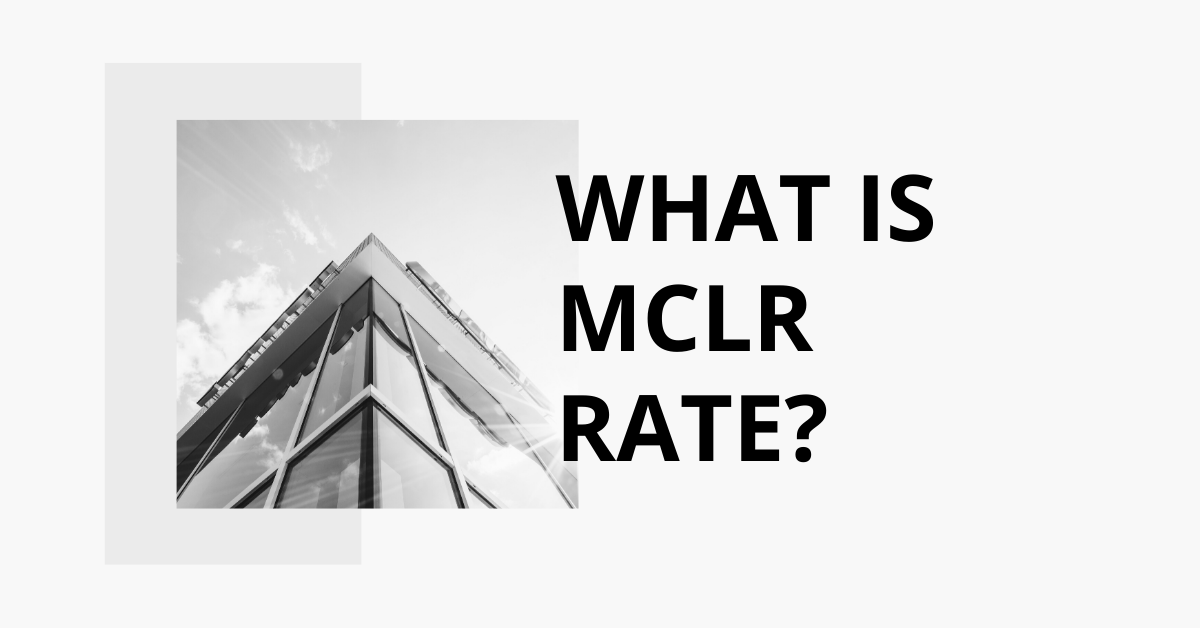# What is Marginal cost of funds-based lending rate (MCLR)?## About Marginal cost of funds-based lending rate:

• It is the minimum interest rate below which no bank is permitted to lend money.
•  It is determined by banks internally, depending upon the loan repayment time.
• The Reserve Bank of India introduced the MCLR methodology for fixing interest rates on 1 April 2016.
•  It replaced the base rate structure, which had been in place since July 2010.
• The rate is determined internally by the bank depending on the period left for the repayment of a loan.
• MCLR is calculated based on four components Marginal cost of fund, Negative carry on account of cash reserve ratio, Operating costs, Tenor premium

### What is the difference between MCLR and base rate

• MCLR is an advanced version of the base rate.
• The base rate is based on the average cost of funds, but MCLR is based on the marginal or incremental cost of money.
•  MCLR depends on the repo rates changed by RBI while Base Rate does not depend on the repo rates changed by RBI.

### Q1) What is base rate?

Base rate is the minimum rate set by the Reserve Bank of India below which banks are not allowed to lend to its customers.